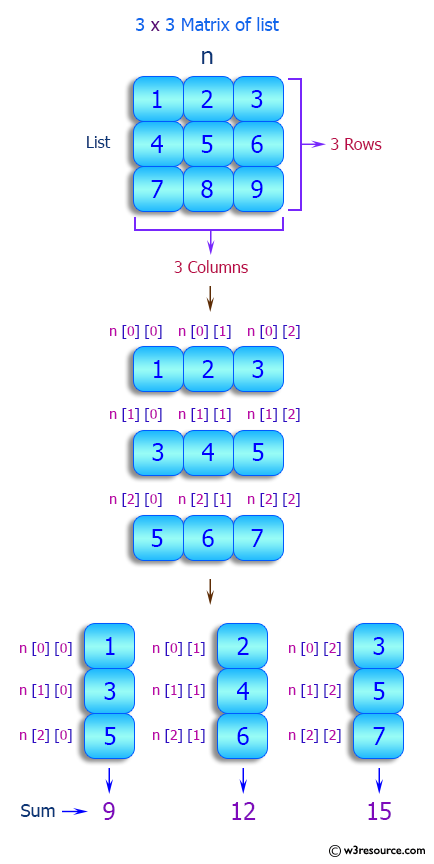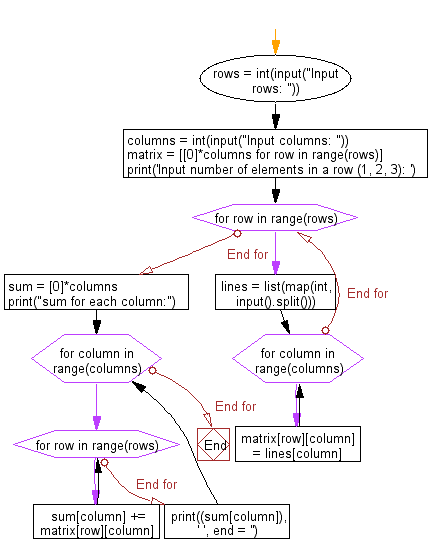﻿ Python: Read a matrix from console and print the sum for each column - w3resource# Python: Read a matrix from console and print the sum for each column

## Python List: Exercise - 87 with Solution

Write a Python program to read a matrix from console and print the sum for each column. Accept matrix rows, columns and elements for each column separated with a space(for every row) as input from the user.

Sample Solution:

Python Code:

``````rows = int(input("Input rows: "))
columns = int(input("Input columns: "))
matrix = [*columns for row in range(rows)]
print('Input number of elements in a row (1, 2, 3): ')
for row in range(rows):
lines = list(map(int, input().split()))
for column in range(columns):
matrix[row][column] = lines[column]

sum = *columns
print("sum for each column:")
for column in range(columns):
for row in range(rows):
sum[column] += matrix[row][column]
print((sum[column]), ' ', end = '')
```
```

Sample Output:

```Input rows:  2
Input columns:  2
Input number of elements in a row (1, 2, 3):
1 2
3 4
sum for each column:
4  6
```

Pictorial Presentation:Flowchart:## Visualize Python code execution:

The following tool visualize what the computer is doing step-by-step as it executes the said program:

Python Code Editor:

Have another way to solve this solution? Contribute your code (and comments) through Disqus.

What is the difficulty level of this exercise?

Test your Python skills with w3resource's quiz

﻿

## Python: Tips of the Day

```print(2_000_000)
```2000000